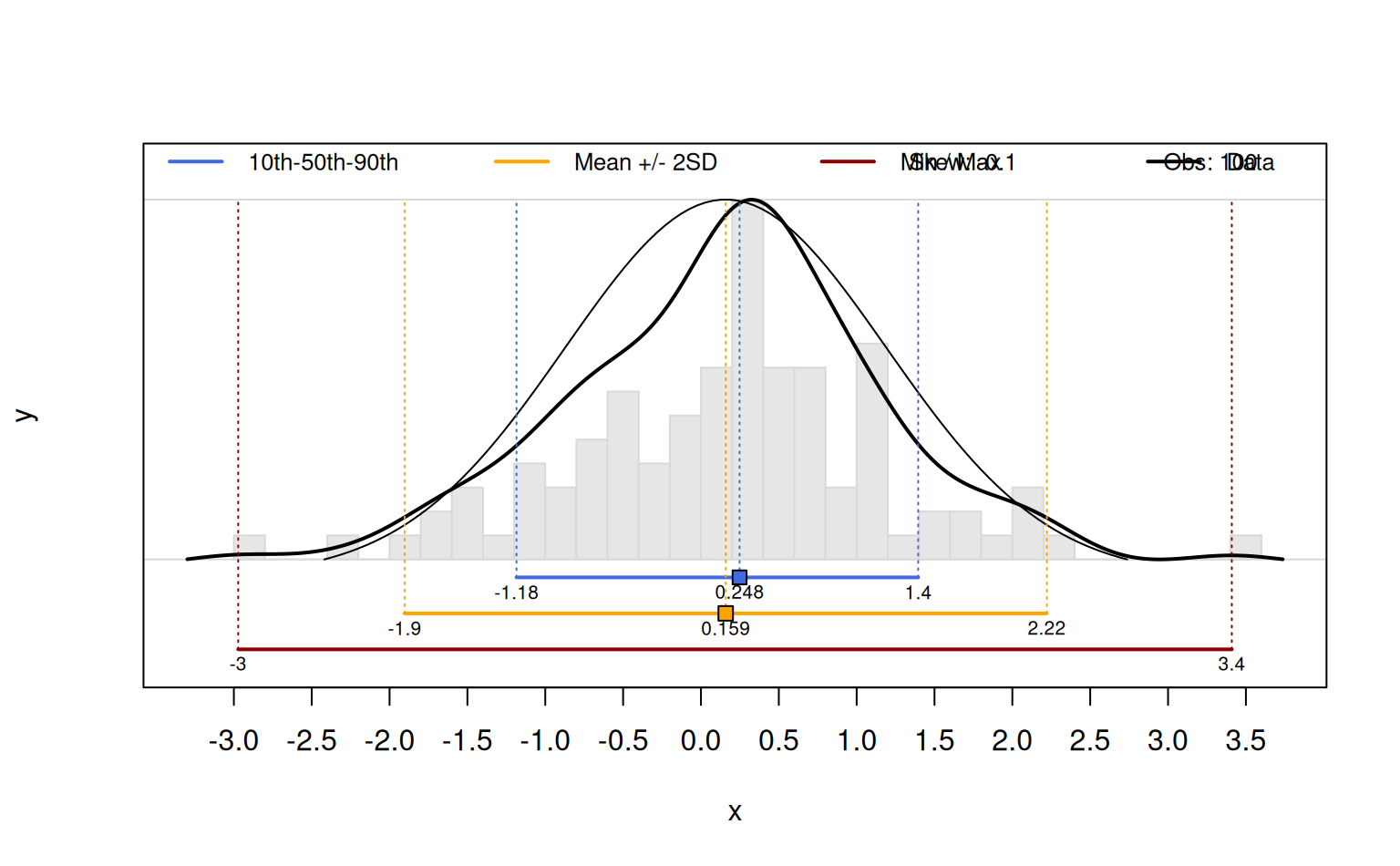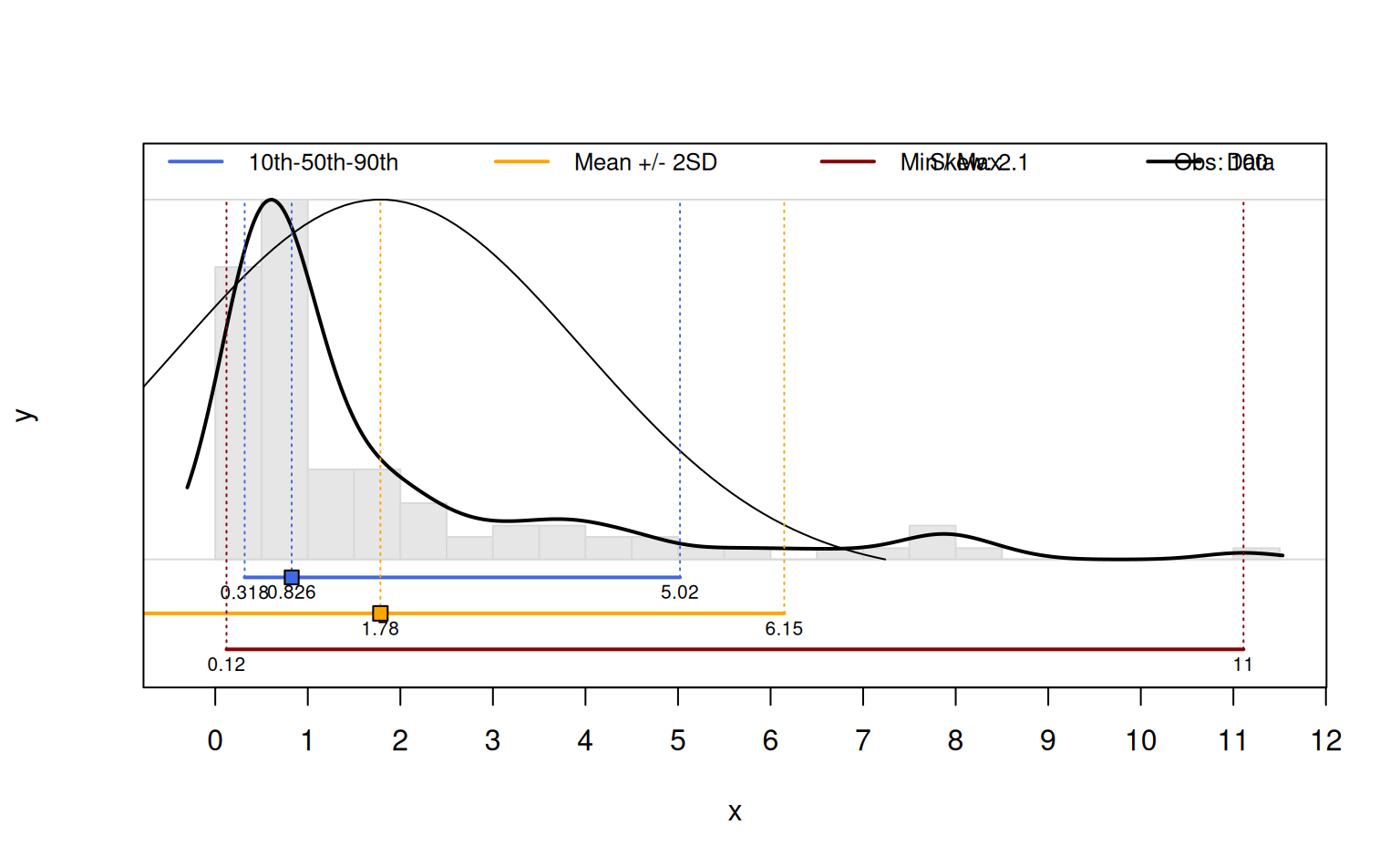This function can be used to graphically demonstrate the relationship between distribution shape, an idealized normal distribution (based on sample mean and sd) shape, and measures of central tendency / spread.

percentileDemo(x, labels.signif = 3, pctile.color = "RoyalBlue",
mean.color = "Orange", range.color = "DarkRed",
hist.breaks = 30, boxp = FALSE, ...)

## Arguments

x

vector of values to summarize

labels.signif

integer, number of significant digits to be used in figure annotation

pctile.color

color used to demonstrate range from 10th to 90th percentiles

mean.color

color used to specify mean +/- 2SD

range.color

color used to specify data range

hist.breaks

integer, number of suggested breaks to hist

boxp

logical, add a box and whisker plot?

...

further arguments to plot

## Value

A 1-row matrix of summary stats is invisibly returned.

D.E. Beaudette

## Note

This function is mainly for educational purposes.

## Examples

x <- rnorm(100)
percentileDemo(x)x <- rlnorm(100)
percentileDemo(x)